# montage

Display multiple image frames as rectangular montage

## Syntax

``montage(I)``
``montage(imagelist)``
``montage(filenames)``
``montage(imds)``
``montage(___,map)``
``montage(___,Name,Value)``
``img = montage(___)``

## Description

example

````montage(I)` displays all frames of a multiframe image array `I`. By default, the `montage` function arranges the images so that they roughly form a square.```
``` `montage(imagelist)` displays a montage of images specified in the cell array `imagelist`. The images can be of different types and sizes.```

example

``` `montage(filenames)` displays a montage of the images with file names specified in `filenames`.```

example

``` `montage(imds)` displays a montage of the images specified in the image datastore `imds`. ```
````montage(___,map)` treats all grayscale and binary images (specified using any of the preceding syntaxes) as indexed images and displays them with the specified colormap `map`. If you specify images using file names or an image datastore, then `map` overrides any internal colormap present in the image files. `montage` does not modify the colormap of RGB images.```

example

````montage(___,Name,Value)` uses name-value pair arguments to customize the display of the image montage.```
````img = montage(___)` returns a handle to the single image object that contains all the frames displayed.```

## Examples

collapse all

`load mristack`

Display the dataset. `montage` treats the data as a multiframe image and displays each slice.

`montage(mristack)`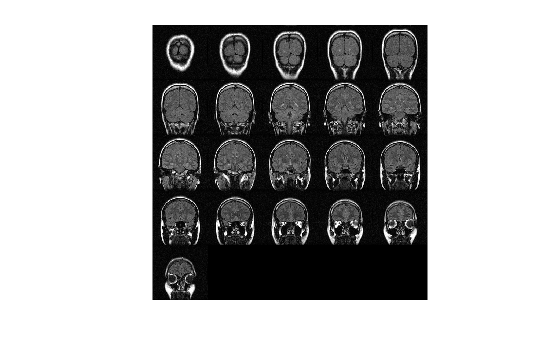Read several images of different types and sizes into the workspace.

```imRGB = imread('peppers.png'); imGray = imread('coins.png');```

Display a montage containing all of the images.

```figure montage({imRGB, imGray, 'cameraman.tif'})```Create a montage from a series of images in files. Make the montage a 2-by-5 rectangle. Then, create a second montage, this time using the `'DisplayRange'` name-value argument to highlight structures in the image.

Display the Images as a Rectangular Montage

Create a string array containing a series of file names.

```fileFolder = fullfile(matlabroot,'toolbox','images','imdata'); dirOutput = dir(fullfile(fileFolder,'AT3_1m4_*.tif')); fileNames = string({dirOutput.name});```

Display the images as a montage. Specify the shape of the montage as a 2-by-5 rectangle.

`montage(fileNames,'Size',[2 5]);`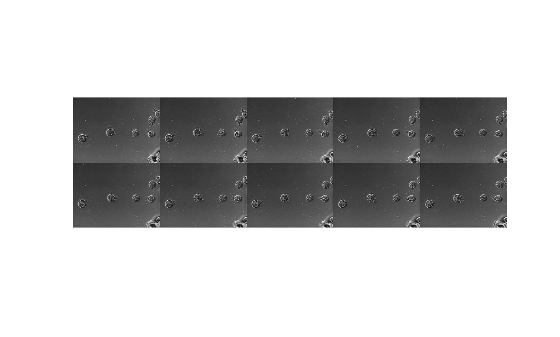Adjust the Contrast of the Images in the Montage

In another figure, create the same 2-by-5 montage. In addition, specify the display range to adjust the contrast of the images in the montage.

```figure montage(fileNames,'Size',[2 5],'DisplayRange',[75 200]);```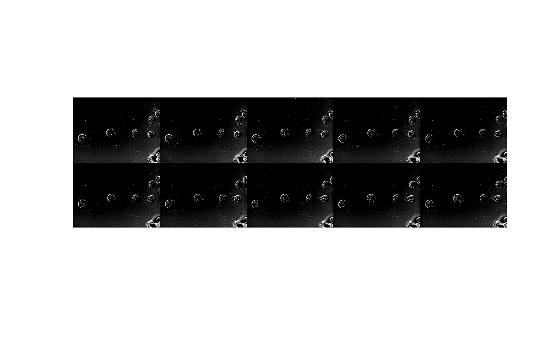View all the images in a MRI data set using montage with default settings. There are 27 images in the set.

```load mri montage(D,map)```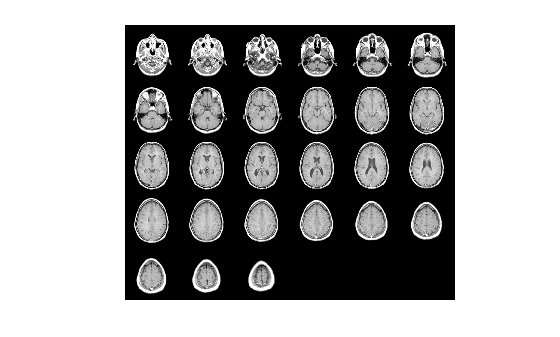Create a new montage containing only the first 9 images.

```figure montage(D,map,Indices=1:9);```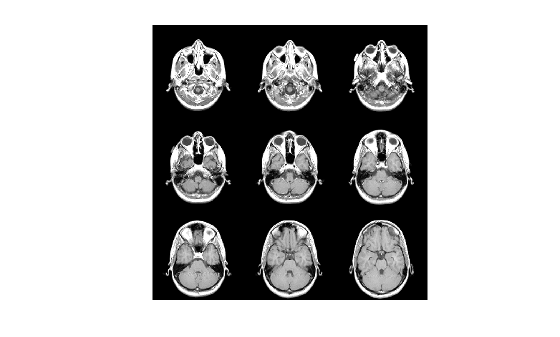Create an `ImageDatastore` object containing a series of ten images from the Image Processing Toolbox™ sample image folder.

```fileFolder = fullfile(matlabroot,'toolbox','images','imdata'); imds = imageDatastore(fullfile(fileFolder,'AT3*'));```

Display the contents of the datastore as a montage.

`montage(imds)`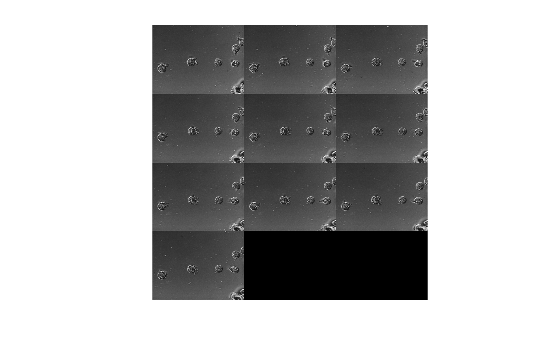## Input Arguments

collapse all

Multiframe image array, specified as one of the following:

• m-by-n-by-k numeric array representing a sequence of k binary or grayscale images

• m-by-n-by-1-by-k numeric array representing a sequence of k binary or grayscale images

• m-by-n-by-3-by-k numeric array representing a sequence of k truecolor images

Data Types: `single` | `double` | `int16` | `uint8` | `uint16` | `logical`

Set of images, specified as a cell array of numeric matrices of size m-by-n or m-by-n-by-3.

Data Types: `single` | `double` | `int16` | `uint8` | `uint16` | `logical` | `cell`

Names of files containing image, specified as a cell array of character vectors or a vector of strings. If the files are not in the current folder or in a folder on the MATLAB® path, then specify the full path name. For more information, see `imread`.

Data Types: `char` | `string` | `cell`

Image datastore, specified as an `ImageDatastore` object.

Colormap, specified as a c-by-3 numeric matrix with values in the range [0, 1]. Each row is a three-element RGB triplet that specifies the red, green, and blue components of a single color of the colormap.

Data Types: `double`

### Name-Value Arguments

Specify optional pairs of arguments as `Name1=Value1,...,NameN=ValueN`, where `Name` is the argument name and `Value` is the corresponding value. Name-value arguments must appear after other arguments, but the order of the pairs does not matter.

Before R2021a, use commas to separate each name and value, and enclose `Name` in quotes.

Example: `'Indices',1:9` creates a montage of the first nine frames

Background color, specified as specified as an RGB triplet, a color name, or a short color name. The `montage` function fills all blank spaces with the background color, including the space specified by `BorderSize`. If you specify a background color, then the `montage` function renders the output as an RGB image.

You can specify any color using an RGB triplet. An RGB triplet is a 3-element row vector whose elements specify the intensities of the red, green, and blue components of the color. The intensities must be in the range [0,1].

You can specify some common colors by name as a string scalar or character vector. This table lists the named color options and the equivalent RGB triplets.

Color NameShort NameRGB TripletAppearance
`'red'``'r'``[1 0 0]``'green'``'g'``[0 1 0]``'blue'``'b'``[0 0 1]``'cyan'` `'c'``[0 1 1]``'magenta'``'m'``[1 0 1]``'yellow'``'y'``[1 1 0]``'black'``'k'``[0 0 0]``'white'``'w'``[1 1 1]`Here are the RGB triplets for the default colors that MATLAB uses in many types of plots.

RGB TripletAppearance
`[0 0.4470 0.7410]``[0.8500 0.3250 0.0980]``[0.9290 0.6940 0.1250]``[0.4940 0.1840 0.5560]``[0.4660 0.6740 0.1880]``[0.3010 0.7450 0.9330]``[0.6350 0.0780 0.1840]`Example: `'BackgroundColor','r'`

Example: `'BackgroundColor','green'`

Example: ```'BackgroundColor',[0 0.4470 0.7410]```

Padding around each thumbnail image, in pixels, specified as a nonnegative integer or a 1-by-2 vector of nonnegative integers. The `montage` function pads the image borders with the background color, `BackgroundColor`.

Data Types: `single` | `double` | `int8` | `int16` | `int32` | `int64` | `uint8` | `uint16` | `uint32` | `uint64`

Display range of grayscale images in array `I`, specified as 1-by-2 vector of the form ```[low high]```. All pixel values less than or equal to low display as black. All pixel values greater than or equal to high display as white. If you specify an empty matrix (`[]`), then `montage` uses the minimum and maximum pixel values of the images.

Data Types: `single` | `double` | `int8` | `int16` | `int32` | `int64` | `uint8` | `uint16` | `uint32` | `uint64`

Frames to display in the montage, specified as an array of positive integers. The `montage` function interprets the values as indices into array `I` or into cell array `filenames` or `imagelist`.

By default, the `montage` function displays all frames or image files.

Example: `'Indices',1:4` create a montage of the first four frames in `I`

Example: `'Indices',1:2:20` displays every other frame.

Data Types: `single` | `double` | `int8` | `int16` | `int32` | `int64` | `uint8` | `uint16` | `uint32` | `uint64`

Interpolation technique used when scaling an image, specified as the comma-separated pair consisting of `'Interpolation'` and one of these values.

ValueDescription
`'nearest'`Nearest neighbor interpolation (default)
`'bilinear'`Bilinear interpolation

Parent of the image object created by `montage`, specified as an axes object. The `montage` function resizes the image to fit the extents available in the parent axes.

Number of rows and columns of images, specified as a 2-element vector of the form `[nrows ncols]`.

If you specify `NaN` or `Inf` for a particular dimension, then the `montage` function calculates the value of the dimension to display all images in the montage. For example, if `'Size'` is ```[2 NaN]```, then the montage will have two rows and the minimum number of columns to display all images. When there is a mismatch between `'Size'` and number of images (frames) specified, then the `montage` function creates the tiled image based on `'Size'`.

Data Types: `single` | `double`

Size of each thumbnail, in pixels, specified as a 2-element vector of positive integers. The aspect ratio of each image is preserved, and any blank space is filled with the background color, `BackgroundColor`.

If you specify an empty array (`[]`), then the thumbnail size is the full size of the first image. If you specify either element as `NaN` or `Inf`, then the `montage` function calculates the corresponding value automatically to preserve the aspect ratio of the first image.

Data Types: `single` | `double`

## Output Arguments

collapse all

Montage image, returned as an Image object.

## Tips

• If you specify an indexed image, then `montage` converts it to RGB using the colormap present in the file.

• If there is a data type mismatch between images, then the `montage` function converts all images to data type `double` using the `im2double` function.

• When calculating the number of images to display horizontally and vertically, `montage` considers the aspect ratio of the images, so that the displayed montage is nearly square.

## Version History

Introduced before R2006a